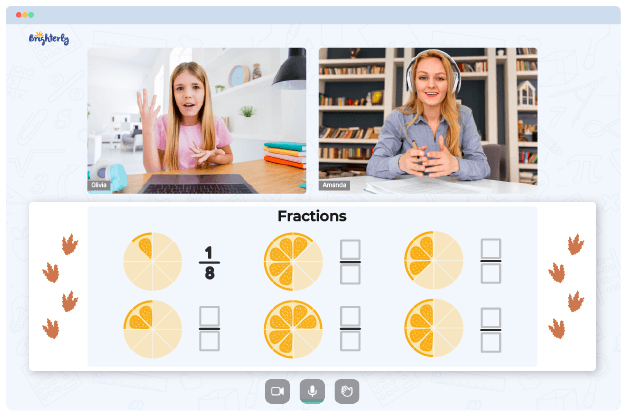The use of the fraction worksheets 6th grade is a seamless way to make your sixth graders master the concepts of fractions. These worksheets comprise questions on various forms of fractions, fraction conversion, and fraction word problems. The worksheets have lots of benefits for students, tutors, and parents.

## Brighterly’s fraction word problems worksheets 6th grade

By learning with the fractions worksheets, students will master mathematical concepts like ratios, proportions, percentages, etc. Top experts with years of experience tutoring kids in mathematics design worksheets. Thus, the sheets are tailored to meet the demands of kids and make learning seamless for them.

Brighterly’s 6th grade fraction to decimal worksheet is suitable for all grade six students, irrespective of their learning capability. The worksheets are deliberately designed to make learning an enjoyable and interactive experience.

Math for Kids

Is Your Child Struggling With Math?
1:1 Online Math TutoringFurthermore, the questions on the worksheets are arranged according to varying difficulty levels. That is, kids proceed from less complicated to more challenging tasks.

## Printable 6th grade fractions worksheets

Printable fractions worksheets for sixth graders make it easier for parents to make their kids study. Since the tools are in paper format, kids would be able to study regularly. Also, the questions on the worksheets come with answers so kids can check the answers by themselves.### 6th Grade Fraction Worksheets PDF### 6th Grade Fraction Worksheets PDF

Fraction Word Problems Worksheets 6th Grade### 6th Grade Fraction Worksheets PDF### 6th Grade Fraction Worksheets PDF

6th Grade Fraction To Decimal Worksheet

### Fractions Worksheets

Need help with Fractions?• Does your child struggle to master fractions?
• Try lessons with an online tutor.

Is your child having trouble grasping the concept of fractions? An online tutor could provide the necessary support.